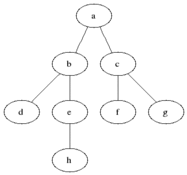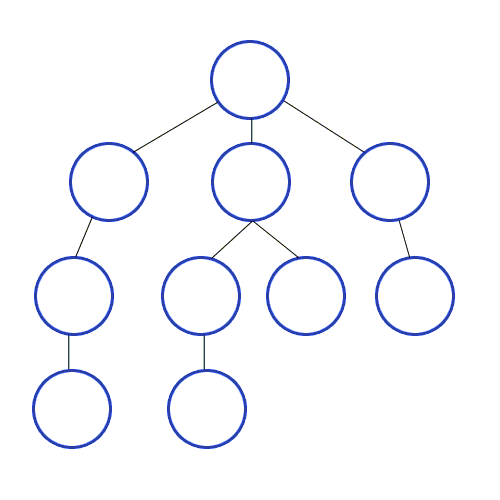# Hacktoberfest 2018 #2: Graph Algorithms Implementation In Swift

October 14, 2018

The second week of Hacktoberfest is almost finished, and this week I’ve made another contribution. I decided to continue developing my skills in Swift programming, and after scrolling through the issues with label “hacktoberfest”, I found a project called “All Algorithms”. The idea of this project is that there are different repositories with names of programming languages, and each of these repositories implements different algorithms in a particular language. I didn’t really do any algorithms and data structures before, so I thought that it would be a great opportunity to work with algorithms by contributing to this project.

Obviously, I chose Swift, and, fortunately, there were not many algorithms developed in Swift repository. After having a quick look, I didn’t find any graph implementations there, so I let maintainers know that I would like to work on the graph algorithms, and I was welcomed.

I decided to add two algorithms to the project:

• Breadth-first search
• Depth-first search

I set up a local repository and started to work on the issue. Unlike the issue from last week, I didn’t have to get familiar with the existing code because I needed to implement the algorithms from scratch.

I started a research by reading 22 chapter of “Introduction to Algorithms” by Cormen, Leiserson, Rivest, and Stein. The chapter is called “Elementary Graph Algorithms”. I read first three parts of it:

1. Representations of graphs 2. Breadth-first search 3. Depth-first search

## Representations of graphs

There are two ways of representing graph in computer: 1. Adjacency-list 2. Adjacency-matrix

Here is an example of graph (a) presented in adjacency-list (b) and adjacency-matrix (c):I found that it’s better to use adjacency-matrix when the graph is dense (many edges), but if a graph is sparse, you’d better use adjacency-list.

An adjacency-matrix is just a 2-dimensional array of size n² (n — quantity of edges), where 1 is used to represent an edge between nodes, and 0 if there is no connection between vertices. If you have sparse graph (not many edges), it means that most of the matrix entries wouldn’t be used, so it’s just a waste of memory, that’s why adjacency list would be a better solution.

I’ve used adjacency-matrix before, so I decided to use adjacency-list in my graphs for this project.

## Graph implementation in Swift

In order to implement graph data structure, I created three classes:

• Node
• Edge
• Graph

### Node class

``````import Foundation

public class Node: Equatable{
public var label: String
public var neighbors: Array<Edge>
public var color: String?
public var predecessor: Node?
public var distance: Int?

public init(_ label: String){
self.label = label
self.neighbors = Array<Edge>()
}

public static func == (lhs: Node, rhs: Node) -> Bool{
return lhs.label == rhs.label
}
}``````

### Edge class

``````import Foundation

public class Edge {
public var neighbor: Node
public var weight: Int?

public init(_ neighbor: Node){
self.neighbor = neighbor
}
}``````

### Graph class

``````import Foundation

public class Graph{
public var nodes: Array<Node>

public init(){
self.nodes = Array<Node>()
}

public func addNode(_ label: String){
let newNode = Node(label)
nodes.append(newNode)
}

public func addEdge(from source: Node, to destination: Node){
let newEdge = Edge(destination)
source.neighbors.append(newEdge)
destination.neighbors.append(Edge(source))
}
}``````

## Algorithms

### Breadth-first searchBreadth-first search (BFS) starts from a specified source node, and explores its neighbours by discovering all nodes on the same level, and then going to the next depth level (see animation above).

This algorithm uses coloring to keep track of progress, so that

• White color stands for undiscovered vertex
• Gray color stands for discovered vertex
• Black color stands for visited vertex

Here is the pseudocode of the algorithm (from: Introduction to Algorithms):

``````BFS(G, s)
for each vertex u ∈ G.V - {s}
u.color = WHITE
u.d = ∞
u.π = NIL

s.color = GRAY
s.d = 0
s.π = NIL
Q = Ø

ENQUEUE(Q, s)

while Q ≠ Ø
u = DEQUEUE(Q)

for each v ∈ G.Adj[u]
if v.color == WHITE
v.color = GRAY
v.d = u.d + 1
v.π = u

ENQUEUE(Q, v)

u.color = BLACK``````

where d — distance from source node, π — predecessor(parent node), s — source node.

As you can see, this pseudocode uses Queue data structure, so I developed a Queue struct:

``````import Foundation

public struct Queue<T> {
var items: [T] = []

public init(){}

public mutating func enqueue(element: T){
items.append(element)
}

public mutating func dequeue() -> T? {
if items.isEmpty {
return nil
} else{
return items.remove(at: 0)
}
}

public var isEmpty: Bool {
return items.isEmpty
}
}``````

And here is my implementation of Breadth-first search in Swift:

``````func breadthFirstSearch(graph: Graph, source: Node){
var visitedNodes = Queue<Node>()

for node in graph.nodes{
node.color = "White"
node.distance = nil
node.predecessor = nil
}

source.color = "Gray"
source.distance = 0
source.predecessor = nil

visitedNodes.enqueue(element: source)

while !visitedNodes.isEmpty{
if let currentNode = visitedNodes.dequeue(){
for edge in currentNode.neighbors {
if edge.neighbor.color == "White" {
edge.neighbor.color = "Gray"
edge.neighbor.distance = currentNode.distance! + 1
edge.neighbor.predecessor = currentNode

visitedNodes.enqueue(element: edge.neighbor)
}
}

currentNode.color = "Black"
}
}
}``````

### Depth-first searchDepth-first search (DFS) works in the way it goes down the edges of the most recently discovered node. Once it discovered all the edges of one node, it comes back, and does the same thing with next undiscovered node (see animation above).

In a similar way as BFS, DFS also uses coloring to keep track of progress.

Here is the pseudocode of the algorithm (from: Introduction to Algorithms):

``````DFS (G)
for each vertex u ∈ G.V
u.color = WHITE
u.π = NIL
time = 0

for each vertex u ∈ G.V
if u.color == WHITE
DFS-VISIT(G, u)

DFS-VISIT(G, u)
time = time + 1
u.d = time
u.color = GRAY

for each v ∈ G.Adj[u]
if v.color == WHITE
v.π = u
DFS-VISIT(G, v)

u.color = BLACK
time = time + 1
u.f = time``````

where π — predecessor, time — timestamp, d — timestamp to record when node was discovered, f — timestamp to record when node was visited

Here is my implementation of Depth-first search in Swift:

``````func depthFirstSearch(graph: Graph){
for node in graph.nodes{
node.color = "White"
node.predecessor = nil
}
var timestamp = 0

for node in graph.nodes{
if node.color == "White"{
dfsVisit(node: node, timestamp: &timestamp)
}
}
}

func dfsVisit(node: Node, timestamp: inout Int){
timestamp = timestamp + 1
node.discoveredTimestamp = timestamp
node.color = "Gray"

for edge in node.neighbors{
if edge.neighbor.color == "White"{
edge.neighbor.predecessor = node
dfsVisit(node: edge.neighbor, timestamp: &timestamp)
}
}

node.color = "Black"
timestamp = timestamp + 1
node.finishedTimestamp = timestamp
}``````

You can see the source code in my Pull Request, where I also created a sample graph and ran BFS and DFS. To sum up this week with some outcomes:

• implemented Graph in Swift
• studied and implemented Breadth-search algorithm
• studied and implemented Depth-search algorithm
• strengthened my skill in Swift development